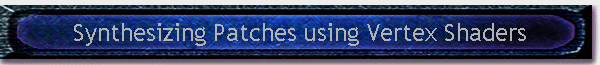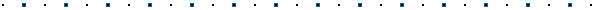#Written by Robert Dunlop Microsoft DirectX MVP< Previous: Page 3 Page 4 Next: Page 5 >

## Measuring Height at Arbitrary Locations

For purposes such as collision detection and terrain following, it is necessary to be able to determine the height (Y) for a given point (X,Z) on the terrain.  Rather than having to perform a mesh intersection test, getting the height at an arbitrary point is simply a matter of determining which quad the point is in, then calculating the height at that point in the quad:

 See Listing 4 for the CalcQuad() function called in this listing float CAppForm::CalcHeight(float x, float z) {     // offset to base     x-=m_vQuadBase.x;     z-=m_vQuadBase.z;     // normalize to quad size     x/=m_fQuadSize;     z/=m_fQuadSize;     // get integer part     int row=(int) z;     int col=(int) x;     // return if out of range     if (row<0||row>=m_numSplines-3||col<0||col>=m_numSplines-3) {         backCol=0x00ffff00;         return 0.0f;     }     // get fractional parts     x-=floorf(x);     z-=floorf(z);     // calculate and return quad height     return m_vQuadScaling.y            * CalcQuad(x,z,&splineMat[row][col])            + m_vQuadBase.y; } Listing 10

## Rendering the Landscape

Before rendering the landscape, we will first perform the following initializations:Set up D3D to render from our vertex and index buffersSet D3D to use our programmable vertex shaderCombine and transpose the world, view, and projection matrices and set as a vertex shader constantSet a vertex shader constant to a vector defining the patch scaling.

These steps are shown in Listing 11, below:

 m_pd3dDevice->SetIndices(m_pIB,0); m_pd3dDevice->SetStreamSource( 0, m_pVB, sizeof(SPLINEVERTEX) ); m_pd3dDevice->SetVertexShader(m_hVertexShader); D3DXMATRIX mat,matViewWorld; D3DXMatrixMultiply( &matViewWorld, &m_matWorld, &m_matView); D3DXMatrixMultiply( &mat, &matViewWorld, &m_matProj); D3DXMatrixTranspose( &mat, &mat ); // shader input : c0-c3 = world/view/proj matrix m_pd3dDevice->SetVertexShaderConstant( 0, &mat, 4 ); // shader input : c4 = patch scaling m_pd3dDevice->SetVertexShaderConstant( 4, &m_vQuadScaling, 1 ); Listing 11

The landscape is then rendered by repeatedly drawing the same vertex buffer, applying different vertex shader constants each time to set the patch shape, lighting, and location:

 // loop through the patches for (int i=0;iSetVertexShaderConstant(5,                                               &(D3DXVECTOR4(baseX,                                                             m_vQuadBase.y,                                                             baseZ,                                                             0.0f)),                                               1 );         // shader input : c6-c9 = patch altitude matrix         m_pd3dDevice->SetVertexShaderConstant( 6, &splineMat[i][j], 4 );         // shader input : c16-c19 = patch lighting matrix         m_pd3dDevice->SetVertexShaderConstant( 16, &splineNormMat[i][j], 4 );         // render the patch         m_pd3dDevice->DrawIndexedPrimitive( D3DPT_TRIANGLELIST,                                             0,                                             m_patchWidth*m_patchWidth,                                             0,                                             (m_patchWidth-1)*(m_patchWidth-1)*2);     } } Listing 12
 < Previous: Page 3 Page 4 Next: Page 5 >Table of Contents

Page 1

Page 2 Page 3

Introduction

Streamlining the calculations Computing Vertex Position

Catmull-Rom Splines

Using Vertex Shaders to Render Patches Computing Vertex Color

Surface Representation with Splines

Storage of Common Quad Data

The Completed Vertex Shader
Page 4 Page 5
Setting Up the Landscape The Demo Application
Measuring Height at Arbitrary Locations Potential Improvements
Rendering the LandscapeThis site, created by DirectX MVP Robert Dunlop and aided by the work of other volunteers, provides a free on-line resource for DirectX programmers.

Special thanks to WWW.MVPS.ORG, for providing a permanent home for this site.

#####Visitors Since 1/1/2000:Last updated: 07/26/05.